# NCERT Solutions for Class 11 Chemistry Equilibrium Part-1## myCBSEguide App

Complete Guide for CBSE Students

NCERT Solutions, NCERT Exemplars, Revison Notes, Free Videos, CBSE Papers, MCQ Tests & more.

NCERT solutions for Class 11 Chemistry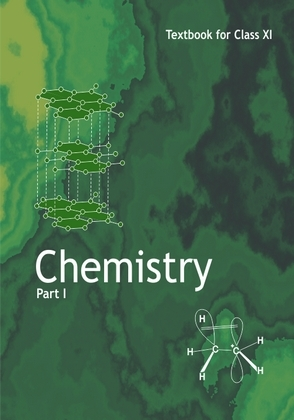## NCERT Class 11 Chemistry Chapter wise Solutions

• 1 – Some Basic Concepts of Chemistry
• 2 – Structure of Atom
• 3 – Classification of Elements and Periodicity in Properties
• 4 – Chemical Bonding and Molecular Structure
• 5 – States of Matter
• 6 – Thermodynamics
• 7 – Equilibrium
• 8 – Redox Reactions
• 9 – Hydrogen
• 10 – The s-Block Elements
• 11 – The p-Block Elements
• 12 – Organic Chemistry – Some Basic Principles and Techniques
• 13 – Hydrocarbons
• 14 – Environmental Chemistry

## NCERT Solutions for Class 11 Chemistry Equilibrium Part-1

1. A liquid is in equilibrium with its vapour in a sealed container at a fixed temperature. The volume of the container is suddenly increased.

a) What is the initial effect of the change on vapour pressure?

b) How do rates of evaporation and condensation change initially?

c) What happens when equilibrium is restored finally and what will be the final vapour pressure?

2. What is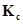for the following equilibrium when the equilibrium concentration of each substance is: [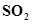]= 0.60 M, [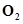] = 0.82 M and [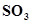] = 1.90 M ?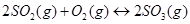3. At a certain temperature and total pressure of 105 Pa, iodine vapour contains 40% by volume of I atoms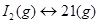Calculate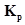for the equilibrium.

4. Write the expression for the equilibrium constant, Kc for each of the following reactions: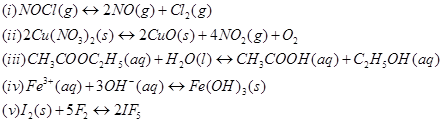5. Find out the value of Kc for each of the following equilibria from the value of Kp: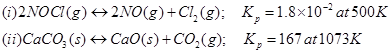6. For the following equilibrium,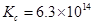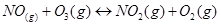Both the forward and reverse reactions in the equilibrium are elementary bimolecular reactions. What is, for the reverse reaction?

7. Explain why pure liquids and solids can be ignored while writing the equilibrium constant expression?

8. Reaction between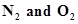takes place as follows: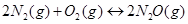If a mixture of 0.482 mol of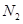and 0.933 mol of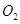is placed in a 10 L reaction vessel and allowed to form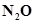at a temperature for which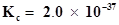, determine the composition of equilibrium mixture.

9. Nitric oxide reacts with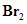and gives nitrosyl bromide as per reaction given below: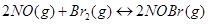When 0.087 mol of NO and 0.0437 mol ofare mixed in a closed container at constant temperature, 0.0518 mol of NO Br is obtained at equilibrium. Calculate equilibrium amount of NO and.

10. At 450 K,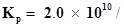bar for the given reaction at equilibrium.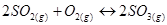What isat this temperature?

11. A sample of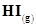is placed in flask at a pressure of 0.2 atm. At equilibrium the partial pressure of HI(g) is 0.04 atm. What is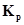for the given equilibrium?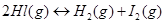12. A mixture of 1.57 mol of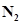, 1.92 mol of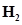and 8.13 mol of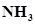is introduced into a 20 L reaction vessel at 500 K. At this temperature, the equilibrium constant,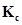for the reaction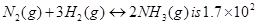Is the reaction mixture at equilibrium? If not, what is the direction of the net reaction?

13. The equilibrium constant expression for a gas reaction is,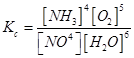Write the balanced chemical equation corresponding to this expression.

14. One mole of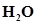and one mole of CO are taken in 10 L vessel and heated to

725 K. At equilibrium 40% of water (by mass) reacts with CO according to the equation,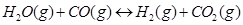Calculate the equilibrium constant for the reaction.

15. At 700 K, equilibrium constant for the reaction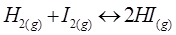is 54.8. If 0.5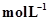of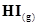is present at equilibrium at 700 K, what are the concentration of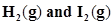assuming that we initially started withand allowed it to reach equilibrium at 700 K?

16. What is the equilibrium concentration of each of the substances in the equilibrium when the initial concentration of ICl was 0.78 M?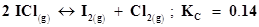17.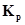= 0.04 atm at 899 K for the equilibrium shown below. What is the equilibrium concentration of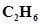when it is placed in a flask at 4.0 atm pressure and allowed to come to equilibrium?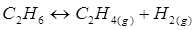18. Ethyl acetate is formed by the reaction between ethanol and acetic acid and the equilibrium is represented as: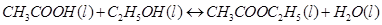(i) Write the concentration ratio (reaction quotient),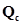, for this reaction (note: water is not in excess and is not a solvent in this reaction)

(ii) At 293 K, if one starts with 1.00 mol of acetic acid and 0.18 mol of ethanol, there is 0.171 mol of ethyl acetate in the final equilibrium mixture. Calculate the equilibrium constant.

(iii) Starting with 0.5 mol of ethanol and 1.0 mol of acetic acid and maintaining it at 293 K, 0.214 mol of ethyl acetate is found after sometime. Has equilibrium been reached?

19. A sample of pure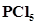was introduced into an evacuated vessel at 473 K. After equilibrium was attained, concentration ofwas found to be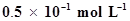. If value ofis 8.3 ×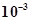, what are the concentrations of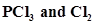at equilibrium?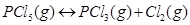20. One of the reactions that takes place in producing steel from iron ore is the reduction of iron (II) oxide by carbon monoxide to give iron metal and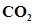.

FeO (s) + CO (g)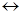Fe (s) +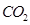(g); Kp= 0.265 at 1050 K.

What are the equilibrium partial pressures of CO andat 1050 K if the initial partial pressures are: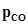= 1.4 atm and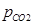= 0.80 atm?

21. Equilibrium constant,for the reaction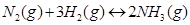at 500 K is 0.061.

At a particular time, the analysis shows that composition of the reaction mixture is 3.0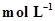, 2.0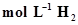and 0.5 mol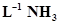. Is the reaction at equilibrium? If not in which direction does the reaction tend to proceed to reach equilibrium?

22. Bromine monochloride, BrCl decomposes into bromine and chlorine and reaches the equilibrium: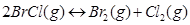for which Kc= 32 at 500 K. If initially pure BrCl is present at a concentration of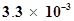, what is its molar concentration in the mixture at equilibrium?

23. At 1127 K and 1 atm pressure, a gaseous mixture of CO andin equilibrium with solid carbon has 90.55% CO by mass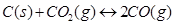. Calculatefor this reaction at the above temperature.

24. Calculate a) ΔG°and b) the equilibrium constant for the formation of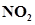from NO and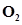at 298 K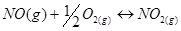Where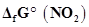= 52.0 kJ/mol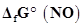= 87.0 kJ/mol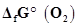= 0 kJ/mol

25. Does the number of moles of reaction products increase, decrease or remain same when each of the following equilibria is subjected to a decrease in pressure by increasing the volume?

(a)(b)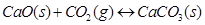(c)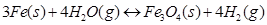## NCERT Solutions for Class 11 Chemistry Chapter 7 Equilibrium

NCERT Solutions for Class 11 Chemistry Equilibrium Part-1 PDF (Download) Free from myCBSEguide app and myCBSEguide website. Ncert solution class 11 Chemistry includes text book solutions from both part 1 and part 2. NCERT Solutions for CBSE Class 11 Chemistry have total 14 chapters. 11 Chemistry NCERT Solutions in PDF for free Download on our website. Ncert Chemistry class 11 solutions PDF and Chemistry ncert class 11 PDF solutions with latest modifications and as per the latest CBSE syllabus are only available in myCBSEguide

## NCERT Solutions for Science Class 3rd to 12th

To download NCERT Solutions for class 11 Chemistry, Physics, Biology, History, Political Science, Economics, Geography, Computer Science, Home Science, Accountancy, Business Studies and Home Science; do check myCBSEguide app or website. myCBSEguide provides sample papers with solution, test papers for chapter-wise practice, NCERT solutions, NCERT Exemplar solutions, quick revision notes for ready reference, CBSE guess papers and CBSE important question papers. Sample Paper all are made available through the best app for CBSE students and myCBSEguide.com website.## Test Generator

Create Papers with your Name & Logo